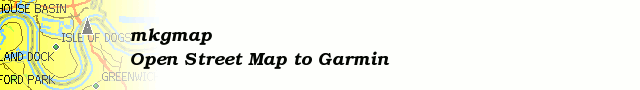# [mkgmap-dev] Overview map: tiny patch/review series

From Mark Burton markb at ordern.com on Tue Jun 16 08:18:09 BST 2009

```Hi Thilo,

> The DP code contains another distance calculation. What you must
> calculate there is the distance of one point to a line. The
> Coord.distance() is used in that formula, but I do not understand the
> formula itself. Maybe it's some genius trick to simplify the
> calculation, but I do not get it. The only thing I see is that when I
> reduce the error distance the simplified ways look "nicer". But the
> preset error distance of one half of the resolution should be small
> enough already. So my assumption is that the actual error distance is
> much larger than expected, which may be due to a bug in the code.

At the distances we're (mostly) interested in, the curvature of the
earth's surface has a tiny effect (much less than the effect of hills &
valleys) so I guess (hope) that leaving out that factor is OK.

I know that this isn't your code but it's as good a place as any
to comment about it: looking at the DP code (for the first time), I
immediately see that the loop that finds the max distance is
(potentially) doing many more sqrts and divisions than it needs to. So
even if the code is correct, it could be somewhat faster which would be
worthwhile given that it gets called for every line. Eg, it could be
changed to look like this:

// Find point with highest distance.
// Loop runs downwards, as the list length gets modified while running
for(int i = endIndex-1; i > startIndex; i--) {
Coord p = points.get(i);
double ap = p.distance(a);
double bp = p.distance(b);
double abpa = (ab+ap+bp)/2;
double dd = abpa * (abpa-ab) * (abpa-ap) * (abpa-bp);
if (dd > maxDistance) {
maxDistance = dd;
maxIndex = i;
}
}
maxDistance = 2 * Math.sqrt(maxDistance) / ab;

Also - you get a division by zero if ab is 0, perhaps adding a test
for that before the loop would be advisable.

Another minor nit - the comment is inaccurate as the list length doesn't change in this loop.

Cheers,

Mark

```